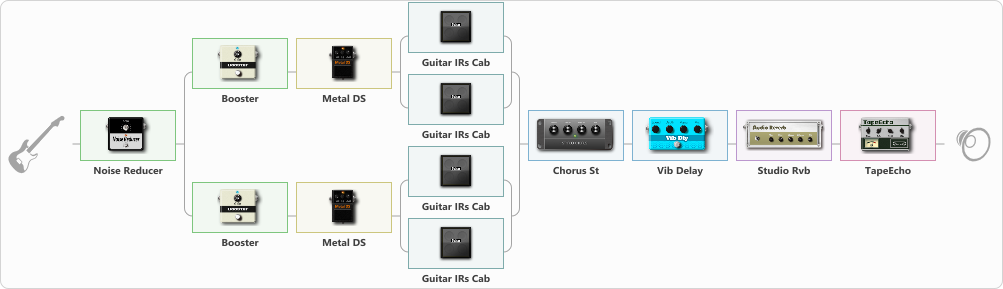# Metal Truss

Discussion in 'ToneLib-GFX presets' started by truss van halen, Apr 9, 2022.

1. ### truss van halenWell-Known Member

Metal Truss

Preset name: BLACK METAL - ATMOS

Effects chain:Effect: "Noise Reducer" (Dynamics / Filter), active - "yes"
{
"Sens" = 69
"Mode" = Soft
}

Effect: "Splitter" (Dynamics / Filter)
{
"A-Bypass" = Off
"A-Pan" = -25
"A-Level" = 55
"B-Bypass" = Off
"B-Pan" = 25
"B-Level" = 55
"Width" = 0

'A' branch:
{

Effect: "Booster" (Dynamics / Filter), active - "yes"
{
"Gain" = 25
}

Effect: "Metal DS" (Overdrive / Distortion), active - "yes"
{
"Dist" = 100
"Bass" = 42
"Middle" = 50
"Treble" = 69
"Level" = 75
}

Effect: "Splitter" (Dynamics / Filter)
{
"A-Bypass" = Off
"A-Pan" = -25
"A-Level" = 55
"B-Bypass" = Off
"B-Pan" = 25
"B-Level" = 55
"Width" = 0

'A' branch:
{

Effect: "Guitar IRs Cab" (Cabinets), active - "yes"
{
"Model" = Orange PPC (4x12")
"Mic Position" = Center
"Mic Distance" = Middle
"Low Cut (Hz)" = 0
"Hi Cut (kHz)" = 20.0
"Mix" = 100
"Level (dB)" = 0
}
}
'B' branch:
{

Effect: "Guitar IRs Cab" (Cabinets), active - "yes"
{
"Model" = Marshall JCM2000 (4x12")
"Mic Position" = Center
"Mic Distance" = Middle
"Low Cut (Hz)" = 0
"Hi Cut (kHz)" = 20.0
"Mix" = 100
"Level (dB)" = 0
}
}
}
}
'B' branch:
{

Effect: "Booster" (Dynamics / Filter), active - "yes"
{
"Gain" = 51
}

Effect: "Metal DS" (Overdrive / Distortion), active - "yes"
{
"Dist" = 100
"Bass" = 74
"Middle" = 50
"Treble" = 69
"Level" = 57
}

Effect: "Splitter" (Dynamics / Filter)
{
"A-Bypass" = Off
"A-Pan" = -25
"A-Level" = 55
"B-Bypass" = Off
"B-Pan" = 25
"B-Level" = 55
"Width" = 0

'A' branch:
{

Effect: "Guitar IRs Cab" (Cabinets), active - "yes"
{
"Model" = Marshall 1960b (4x12")
"Mic Position" = Center
"Mic Distance" = Middle
"Low Cut (Hz)" = 133
"Hi Cut (kHz)" = 8.5
"Mix" = 100
"Level (dB)" = 8
}
}
'B' branch:
{

Effect: "Guitar IRs Cab" (Cabinets), active - "yes"
{
"Model" = Marshall 1960A (4x12")
"Mic Position" = Center
"Mic Distance" = Middle
"Low Cut (Hz)" = 0
"Hi Cut (kHz)" = 20.0
"Mix" = 100
"Level (dB)" = 0
}
}
}
}
}

Effect: "Chorus St" (Modulation / Sfx), active - "yes"
{
"Speed" = 2.8
"Depth" = 58
"Center" = 6.1
"Mix" = 36
}

Effect: "Vib Delay" (Modulation / Sfx), active - "yes"
{
"Speed" = 3.2
"Depth" = 0
"Feedback" = 0
"Time" = 2.6
"Mix" = 100
"Mode" = 2
}

Effect: "Studio Rvb" (Reverberation), active - "yes"
{
"Time" = 4.0
"PreDelay" = 32
"LoDamp" = 0
"HiDamp" = 12
"Mix" = 36
}

Effect: "TapeEcho" (Delay), active - "yes"
{
"Time" = 316
"Feedback" = 23
"Tone" = 79
"LoDamp" = 19
"Mix" = 71
}

Note: You will need to download and install the ToneLib-GFX software to use the preset.

File size:
1.5 KB
Views:
2,211